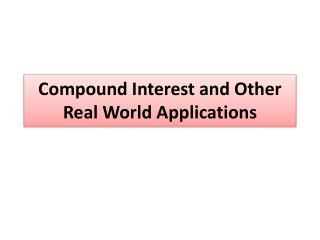DownloadDownload PresentationCompound Interest and Other Real World Applications

# Compound Interest and Other Real World Applications

Télécharger la présentation## Compound Interest and Other Real World Applications

- - - - - - - - - - - - - - - - - - - - - - - - - - - E N D - - - - - - - - - - - - - - - - - - - - - - - - - - -
##### Presentation Transcript

1. Compound Interest and Other Real World Applications

2. What is Compound Interest? When you invest your money in a bank or investment fund, the bank uses the exponential growth to make you money. P: Principle (Amount deposited in the account) r: Rate (or interest rate, growth factor) n: number of times compounded per year t: time (in years) A: amount in the account after t years

3. What Does “Number of Times Compounded” look like? Annually: Means n=1 Semiannually: n=2 Quarterly: n=4 Daily: n=?

4. Ex 1: You deposit \$1,500 in an account that pays 3.5% annual interest. Find the balance after 20 years if the account is compounded semiannually. P = \$1,500 r = .035 t = 20 n = 2

5. Exponential Decay Models There isn’t a big difference between Compound Interest and Exponential Decay Models… P: Initial Amount (the amount started with) r: percent decrease ( is the decay factor) n: number of times compounded per year t: time (in years) A: amount after t years

6. Ex 2: A used Corvette was valued at \$13,000 when it sold. It decreases in value by 15% each year. a. Write an exponential decay model for the value of the car. P = 13,000 r = .15 n = 1

7. Ex 2: A used Corvette was valued at \$13,000 when it sold. It decreases in value by 15% each year. b. Use the model to estimate the value after 5 years. t = 5

8. Ex 2: A used Corvette was valued at \$13,000 when it sold. It decreases in value by 15% each year. c. Use the model to estimate when the car will be worth \$2,000. \$5,768.17 A = 2,000 10 \$2559.37 In about 11 years the car will be worth about \$2,000 11 \$2,175.46

9. Ex 2: A used Corvette was valued at \$13,000 when it sold. It decreases in value by 15% each year. d. Find the annual percent decrease and growth factor. Annual % decrease = 15% Growth Factor: .85

10. Homework: pg.470#46-54pg. 478#46-52